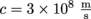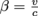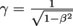Study Guide

# Special Relativity Terms

## Special Relativity Terms

### Clock

Absolutely any object which marks the passing of time in regular intervals.

### Speed of Light

Exactly what it sounds like: the speed at which light particles, called photons, travel. It's universal for any light particle; always equal to.Nothing can go faster than the speed of light, according to the famous Albert Einstein. It's the unbreakable speed limit of the universe.

### Beta, β

The objective judge that compares an object's speed to the speed of light. Everyone is jealous at how fast a photon can go. Judge Beta makes a fair verdict by dividing velocity v by the speed of light c for comparison purposes. In other words,### Frame of Reference

The position and speed from which an event is viewed. If we run down an alley with a camcorder on our heads, it records the side of the alley "running" the opposite way. If this same camcorder is fastened on a trashcan in the same all for the same run it videotapes us whizzing past it for a brief amount of time. A thug chasing after us with his own camcorder sees things happening from his angle, with us (hopefully) an unchanging distance in front of him while the sides of the alley rush backward. These are all different frames of references.

### Inertial Frame of Reference

A frame of reference describing time and space as if space, such as Mother Earth, is independent of Father Time. All of Newtonian physics happens within an inertial frame. Inertial frames have constant velocities. Acceleration creates a non-inertial frame.

### Galilean Transformations

Equations that switch from one inertial frame of reference to another.

### Spacetime Coordinates

Rendezvous coordinates. If we arrange to meet up with a friend somewhere, we agree on a a location and time, given the variable s = (x, y, z, t).

### Event

A set of outcomes of an experiment.

### Relativistic

Think of it as relightivistic. It describes anything moving at a significant fraction of the speed of light.

### Relativistic Factor, γ

Cousins with Judge Beta, Judge Gamma γ compares any property measured in two different frames of references, one of which is moving at a significant fraction of the speed of light,### Proper Time

The time measured for an event at rest in its own frame of reference. It's a possessive thing: the time that belongs to an event as it views itself.

### Time Dilation

The stretching of time by moving at speeds near the speed of light, relative to an observer. How's that for head-scratching?

### Relativistic Time

It should be called improper time. Time measured by a moving frame of reference rather than one at rest. How rude.

### Simultaneous Events

Two or more events occurring simultaneously, or at the same exact time each with respect to its own frame of reference. Note that an event appearing simultaneous is dependent on the frame of reference with which it's viewed. Yes, simultaneity is relative.

### Proper Length

The cousin of proper time. It's the length measured of an object at rest in its own frame of reference.

### Relativistic Length

The rude cousin of proper length. It's the length measured of an object from a moving frame of reference with respect to the object, or the length measured of a moving object relative to a stationary observer.

### Length Contraction

Think of it as contracting lengthy words, like should not into shouldn't. Objects moving at relativistic speeds shorten as viewed by a nonrelativistic frame of reference. True story. The converse is true as well: a relativistic frame of reference would view a nonrelativistic length as shorter, because of the relativistic difference in speeds of the two frames.

### Invariant Quantity

A quantity that never variesno matter which frame of reference considers it. Namely, the speed of light. There are others, too.

### Space-time Interval

The interval between two events, or Δs. This interval is invariant under Lorentz transformations.

### Spacelike Interval

A nospace interval. It describes two events that can't share the same space because there's not enough of it.

### Timelike Interval

A notime interval. Describes two events that can't share the same time because there's not enough time.

### Lightlike Interval

A light interval. An interval that represents events traveling at the speed of light.

### Mass-Energy Equivalence

Einstein's most famous relation, E = mc2. Any mass can be transformed into energy and vice-versa. It includes both the rest energy from mass and kinetic energy together.

### Relativistic Momentum

The momentum of an object moving at a relativistic speed.

### Relativistic Mass

The mass of an object moving at a relativistic speed.

### Rest Mass

The mass of an object at rest, or as viewed from its own frame of reference, otherwise known as proper mass.

### Relativistic Kinetic Energy

The energy from motion of an object moving at a relativistic speed. It's calculated by KE = (γ – 1)mc2.

### Rest Energy

The energy of an object associated with its rest mass through Eo = moc2.

### Lorentz-Einstein Transformations

Equations that switch times and distances from one frame of reference to another, where one at least one frame is moving at a relativistic speed.